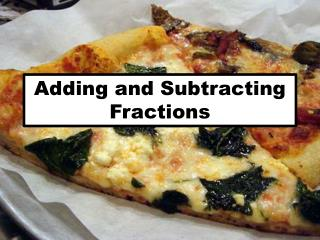DownloadDownload PresentationTélécharger la présentation- - - - - - - - - - - - - - - - - - - - - - - - - - - E N D - - - - - - - - - - - - - - - - - - - - - - - - - - -
##### Presentation Transcript

2. Warm up Find a the missing numbers What is the lowest common multiple of: 2 6 = 3 and 4 12 15 5 5 and 6 30 3 15 = 8 4 and 6 12 40 3 and 8 24 25 5 = 6 5 and 10 10 30

3. Adding and Subtracting Fractions Two rulers add three rulers is: 5 rulers 9 pens Four pensadd fivepens is: Two pens add Five rulers is: 7 Pieces of equipment We can’t so this until we have a name that describes both groups When we add fractions we need them to have the same name

4. Adding and Subtracting Fractions = 5 7 2 7 + 3 7 = 6 9 2 9 + 4 9 = 4 11 1 11 + 3 11

5. Adding and Subtracting Fractions = 5 7 2 7 + 3 7 = 6 9 2 9 + 4 9 = 2 11 5 11 - 3 11

6. Adding and Subtracting Fractions We need to make sure the denominator of both fractions is the same before we add or subtract We need a number in the 5 and 10 times table to use as our bottom number- 10? = 2 5 + 3 10 4 10 + 3 10 = 7 10

7. Adding and Subtracting Fractions We need to make sure the denominator of both fractions is the same before we add or subtract We need a number in the 3 and 12 times table to use as our bottom number- 12? = 1 3 + 5 12 4 12 + 5 12 = 9 12

8. Adding and Subtracting Fractions We need to make sure the denominator of both fractions is the same before we add or subtract We need a number in the 5 and 6 times table to use as our bottom number- 30? = 2 5 + 3 6 12 15 30 + 30 27 30 =

9. Adding and Subtracting Fractions We need to make sure the denominator of both fractions is the same before we add or subtract We need a number in the 8 and 3 times table to use as our bottom number- 24? = 7 8 - 2 3 21 16 24 - 24 = 5 24

10. 7/8 • 8/12 • 7/9 • 7/10 • 2/3 • 11/12 • 7/10 • 8/15 • 16/12 or 1 ¼ • 11/24 • 1 7/12 • 1 5/56 • 29/30 • 29/52 • 1 1/10 • 5/8 • 5/12 • 1/3 • 2/5 • 1/2 • 7/20 • 8/21 • 23/36 • 9/22 • 71/126 Adding and Subtracting Fractions Click for answers